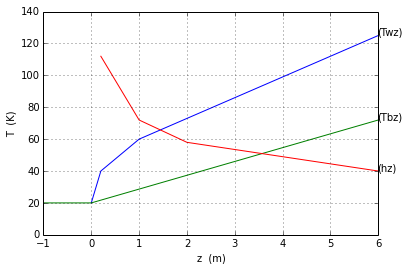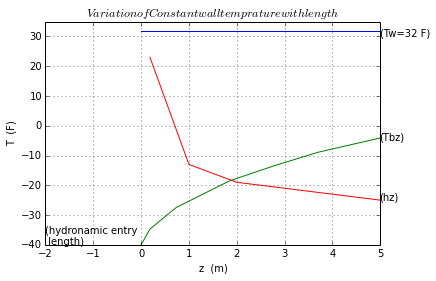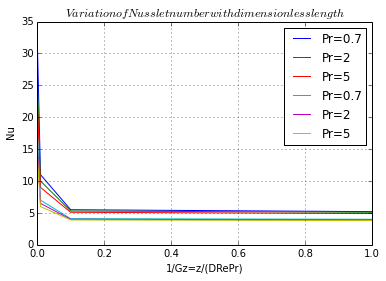# Chapter 6: Convection Heat Transfer in a Closed Circuit¶

### Example 6.1 page No.301¶

In :
Cp_20=2382
rou_20=1.116*1000
v_20=19.18e-6
kf_20=0.249
a_20=0.939e-7
Pr_20=204.0
OD=1.588/100.0
ID=1.446/100.0
A=1.642e-4
Q=3.25e-6

V=Q/A
Re=V*ID/v_20
Z_h=0.05*ID*Re
Tbi=20 # bulk-fluid inlet temperature in degree celsius
qw=2200 # incident heat flux in W/m**2
L=3 # Length of copper tube in m
R=ID/2 # inner radius in m
Tbo=Tbi+(2*qw*a_20*L)/(V*kf_20*R)
Z_t=0.05*ID*Re*Pr_20
Two=Tbo+(11*qw*ID)/(48*kf_20) # The wall temperature at outlet in degree celsius

print"The bulk-fluid outlet temperature is  degree celsius",round(Tbo,0),"C"
print"The wall temperature at outlet is  degree celsius",round(Two,0),"C"

The bulk-fluid outlet temperature is  degree celsius 55.0 C
The wall temperature at outlet is  degree celsius 84.0 C


### Example 6.2 page No.308¶

In :
T_avg=(140+70)/2.0
rou=0.994*62.4
kf=0.363
cp=0.9980
a=5.86e-3
v=0.708e-5
Pr=4.34
OD=1.125/12.0   # outer diameter in ft
ID=0.8792     # inner diameter in ft
A=0.006071    # cross sectional area in sq.ft
m_flow=1.5    # mass flow rate in lbm/s
V=m_flow*3600.0/(rou*A); # velocity in ft/hr
import math
L=20.0
Tw=240.0
Tbo=140.0
Tbi=70.0
hL=-(rou*V*ID*cp*math.log((Tw-Tbo)/(Tw-Tbi)))/(4*L)

print"The average convective coefficient is ",round(hL/10,1),"BTU/(hr. sq.ft.degree Rankine"

The velocity is  14340.4425132 ft/hr
The average convective coefficient is  517.7 BTU/(hr. sq.ft.degree Rankine

In [ ]:
%matplotlib inline


### Example 6.3 page No. 310¶

In :
kf=0.6         # thermal conductivity in W/(m-K)
cp=3.85*1000   # specific heat in J/(kg*K)
rou=1030      # density in kg/m**3
mu=2.12e3     # viscosity in N s/m**2
OD=1.588/100   # outer diameter in m
ID=1.340/100   # inner diameter in m
A=1.410e-4     # cross sectional area in m**2
rou=1030
V=0.1
mu=2.12e-3

Re=rou*V*ID/(mu)
ze=0.05*ID*Re
Tbo=71.7 # final temperature in degree celsius
Tbi=20 # initial temperature in degree celsius
L=6 # heating length in m
qw=rou*V*ID*cp*(Tbo-Tbi)/(4*L)
q=qw*math.pi*ID*L
Pr=(cp*mu)/kf # Prandtl Number
zf=0.05*ID*Re*Pr

print"The heat flux is ",round(qw,0),"W/sq.m"
print"The power required is ",round(q,0),"W"
print"The length required for flow to be thermally developed is",round(zf,1),"m"
import matplotlib.pyplot as plt
fig = plt.figure()

z1=[0,0.2,1,6]
Twz=[20,40,60,125]
z2=[-1,0,6]
Twb=[20,20,72]
z3=[0.2,1,2,6]
hz=[112,72,58,40]
plt.grid()
xlabel("z  (m)")
ylabel("T  (C)  ")
plt.xlim((-1,6))
plt.ylim((0,140))

ax.annotate('(Twz)', xy=(6,125))
ax.annotate('(Tbz)', xy=(6,72))
ax.annotate('(hz)', xy=(6,40))
a1=plot(z1,Twz)
a2=plot(z2,Twb)
a3=plot(z3,hz)
show(a1)
show(a2)
show(a3)

The heat flux is  11447.0 W/sq.m
The power required is  2891.0 W
The length required for flow to be thermally developed is 5.9 m### Example 6.4 page No. 313¶

In [ ]:
%matplotlib inline

In :
kf=0.04       # thermal conductivity in BTU/(hr.ft.°R)
cp=0.2139     # specific heat in BTU/(lbm-°R)
rou= 1.489*(62.4) # density in lbm/cu.ft
v=0.272e-5    #   viscosity in sq.ft/s
a=2.04e-3     # diffusivity in sq.ft/hr
Pr=4.8        # Prandtl Number
OD=0.5/12.0     # outer diameter in ft
ID=0.03350      # inner diameter in ft
A=0.0008814     # cross sectional area in sq.ft
z=5.0
Tw=32.0
Tbo=-4.0
Tbi=-40.0
L=5.0

x=2*a*L/((kf*ID/2.0)*(math.log((Tw-Tbo)/(Tw-Tbi))))   #x=V/hl
V=336.0              #ft/h
V_final=V/3600.0     #ft/s
hl_=V_final/(x)     #

Re=(V_final/3600.0)*ID/v
m_Fr=rou*A*V_final
As=math.pi*ID*L
q=hl_*As*((Tw-Tbo)-(Tw-Tbi))/(log((Tw-Tbo)/(Tw-Tbi)))
q_check=m_Fr*cp*(Tbo-Tbi)
rou_water=1.002*62.4 # density of water in lbm/ft**3 from appendix table C11
m_water=rou_water*L*(2/12.0)*(3/12.0)
t=144*m_water/(-q*3600)

print"The mass flow rate of Freon-12 is ",round(m_Fr*3600,2),"lbm/hr"
print"The required time is ",round(t,0),"hr"

import matplotlib.pyplot as plt
fig = plt.figure()

z1=[0,5]
Tw=[32,32]
z2=[0,0.185,0.74,1.85,2.77,3.70,5.0]
Tbz=[-40,-34.7,-27.4,-18.5,-13.5,-8.9,-4.1]
z3=[0.185,1,2,5.0]
hz=[23,-13,-19,-25]

plt.grid()
xlabel("z  (m)")
ylabel("T  (F)  ")
plt.xlim((-2,5))
plt.ylim((-40,35))

ax.annotate('(Tw=32 F)', xy=(5,30))
ax.annotate('(Tbz)', xy=(5,-5))
ax.annotate('(hz)', xy=(5,-25))
ax.annotate('(hydronamic entry\n length)', xy=(-2,-40))
a1=plot(z1,Tw)
a2=plot(z2,Tbz)
a3=plot(z3,hz)
title('$Variation of Constant wall temprature with length$')
show(a1)
show(a2)
show(a3)

x1=[0.001,0.01,0.1,1]
Nu1=[31,11,5.5,5.2]
Nu2=[25,10,5.3,5.1]
Nu3=[22,9,5.1,4.9]
Nu4=[17,7,4.1,4]
Nu5=[15,6.5,4,3.9]
Nu6=[13.8,6,3.9,3.8]

plt.grid()
xlabel("1/Gz=z/(DRePr)")
ylabel("Nu  ")

plt.xlim((0.001,1))
plt.ylim((0,35))
ax.annotate('(Constant wall temprature)', xy=(0.1,30))
ax.annotate('(Hydronamically and thermally developing laminar flow)', xy=(0.1,25))
b1=plot(x1,Nu1,label='Pr=0.7')
b2=plot(x1,Nu2,label='Pr=2')
b3=plot(x1,Nu3,label='Pr=5')
b4=plot(x1,Nu4,label='Pr=0.7')
b5=plot(x1,Nu5,label='Pr=2')
b6=plot(x1,Nu6,label='Pr=5')
plt.legend(loc='upper right')
title('$Variation of Nusslet number with dimensionless length$')
show(b1)
show(b2)
show(b3)
show(b4)
show(b5)
show(b6)

The mass flow rate of Freon-12 is  27.52 lbm/hr
The required time is  9.0 hr### Example 6.5 page No.320¶

In :
OD = 2.858/100.0     # outer diameter in m
ID = 2.528/100.0     # inner diameter in m
A = 5.019e-4         # cross sectional area in sq.m
Q=80*2.957e-5/120   # The volume flow rate of water (at 20°C) in cu.m/s
p_20= 1.000*1000    # density of water at 20°C in kg/cu.m
p_50= 0.990*(1000)  # density in kg/m3
cp= 4181             # specific heat in J/(kg*K)
v = 0.586e-6         # viscosity in sq.m/s
kf = 0.640           # thermal conductivity in W/(m.K)
a = 1.533e-7         # diffusivity in sq.m/s
Pr = 3.68              # Prandtl number

import math
mass_flow=p_20*Q    # mass flow rate through the tube in kg/s
L=3               #  length of tube in m
As=math.pi*ID*L
Tbo=80           # final temperature in °C
Tbi=20           # initial temperature in °C
qw=mass_flow*cp*(Tbo-Tbi)/(As)
q=qw*As
A=math.pi*(ID/2)**2
print"The power required is",round(q,0),"W"
V=mass_flow/(p_50*A) # average velocity at 50 °C
Re=(V*ID)/v        # Reynold's Number
inv_Gz=L/(Re*ID*Pr) # The inverse Graetz number at tube end, based on 50°C conditions
Nu=6.9 #value of corresponding Nusselts Number from figure 6.12
hz=(Nu*kf)/ID
Two=(qw/hz)+Tbo # The outlet wall temperature in °C
print"\nThe outlet wall temperature is",round(Two,0),"C"

The power required is 4945.0 W

The outlet wall temperature is 199.0 C


### Example 6.6 page No.325¶

In :
p = 0.077             # density in lbm/ft^3
cp = 0.240             # specific heat in BTU/(lbm.degree Rankine)
v = 15.28e-5          # viscosity in ft^2/s
kf = 0.0146           # thermal conductivity in BTU/(hr.ft."R)
a = 0.776              # diffusivity in ft^2/hr
Pr = 0.711            # prandtl number
D=7/12.0               # diameter in ft
L=40                 #  length in ft
Tbo=72                # outlet temperature in degree Fahrenheit
Tbi=45                # inlet temperature in degree Fahrenheit
A=math.pi*(D**2)/4    # cross sectional area of duct in ft^2
rou_o=.0748
V=10                 # average velocity in ft/s
mass_flow=rou_o*A*V

V_avg=mass_flow/(p*A)
Re=(V_avg*D)/v
q=mass_flow*cp*(Tbo-Tbi)
hc=1                 # convection coefficient between the outside duct wall
T_inf=105            # The temperature of attic air surrounding the duct in degree Fahrenheit
hz=(0.023*Re**(0.8)*Pr**0.4)*kf/D
qw=(T_inf-Tbo)/((1/hc)+(1/hz))
Two=qw*(1/hz)+Tbo    # The wall temperature at exit in degree Fahrenheit

print"The heat gained by air is",round(q,3),"BTU"
print"The wall temperature at exit is ",round(Two,1),"F"

The heat gained by air is 1.295 BTU
The wall temperature at exit is  82.1 F

In [ ]: